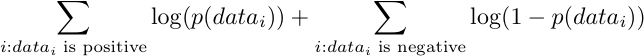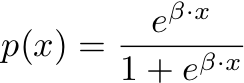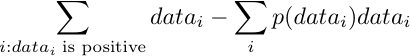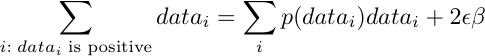# Learn Logistic Regression (and beyond)

One of the current best tools in the machine learning toolbox is the 1930s statistical technique called logistic regression. We explain how to add professional quality logistic regression to your analytic repertoire and describe a bit beyond that.A statistical analyst working on data tends to deliberately start simple move cautiously to more complicated methods. When first encountering data it is best to think in terms of visualization and exploratory data analysis (in the sense of Tukey). But in the end the analyst is expected to deliver actionable decision procedures- not just pretty charts. To get actionable advice the analyst will move up to more complicated tools like pivot tables and Naive Bayes. Once the problem requires control of interactions and dependencies the analyst must move away from these tools and towards the more complicated statistical tools like standard regression, decision trees and logistic regression. Beyond that we have machine learning tools such as: kernel methods, boosting, bagging, decision trees and support vector machines. Which tool is best depends a lot on the situation- and the prepared analyst can quickly try many techniques. Logistic regression is often a winning method and we will use this article to discuss logistic regression a bit deeper. By the end of this writeup you should be able to use standard tools to perform a logistic regression and know some of the limitations you will want to work beyond.

Logistic regression was invented in the late 1930s by statisticians Ronald Fisher and Frank Yates. The definitive resource on this (and other generalized linear models) is Alan Agresti “Categorical Data Analysis” 2002, New York, Wiley-Interscience. Logistic regression is a “categorical” tool in that it is used to predict categories (fraud/not-fraud, good/bad …) instead of numeric scores (like standard regression). For example: consider the “car” data set from the UCI machine learning database ( data, description ). The data is taken from a consumer review of cars. Each car is summarized by 6 attributes ( price, maintenance costs, doors, storage size, seating and safety ); there is also a conclusion column that contains the final overall recommendation (unacceptable, acceptable, good, very good). The machine learning problem is to infer the reviewer’s relative importance or weight of each feature. This could be used to influence a cost constrained design of a future car. This dataset was originally used to demonstrate hierarchical and decision tree based expert systems. But logistic regression can quickly derive interesting results.

Let us perform a complete analysis together (at least in our imaginations if not with our actual computers). First download and install the excellent free analysts workbench called “R”. This software package is an implementation of John Chambers’ S language (a statistical language designed to allow for self-service statistics to relieve some of Chambers’ consulting responsibilities) and a near relative of the SPlus system. This system is powerful and has a number of very good references (our current favorite being Joseph Adler “R in a nutshell” 2009, O’Reilly Media). Using R we can build an interesting model in 2 lines of code.

After installing R start the program and copy the following line into the R command shell.

```CarData <- read.table(url('http://archive.ics.uci.edu/ml/machine-learning-databases/car/car.data'),
```

This has downloaded the car data directly from the UCI database and added a header line so we can refer to variables by name. To see roll-ups or important summaries of our data we could just type in “summary(CarData).” But we will move on with the promised modeling. Now type in the following line:

```logisticModel <- glm(rating!='unacc' ~ buying + maintenance + doors + persons + lug_boot +safety,
```

We now have a complete logistic model. To examine this model we type “summary(logisticModel)”. And see the following (rather intimidating) summary:

```Coefficients:
Estimate Std. Error z value Pr(>|z|)
(Intercept)       -28.4255  1257.5255  -0.023    0.982
buyinglow           5.0481     0.5670   8.904  < 2e-16 ***
buyingmed           3.9218     0.4842   8.100 5.49e-16 ***
buyingvhigh        -2.0662     0.3747  -5.515 3.49e-08 ***
maintenancelow      3.4064     0.4692   7.261 3.86e-13 ***
maintenancemed      3.4064     0.4692   7.261 3.86e-13 ***
maintenancevhigh   -2.8254     0.4145  -6.816 9.36e-12 ***
doors3              1.8556     0.4042   4.591 4.41e-06 ***
doors4              2.4816     0.4278   5.800 6.62e-09 ***
doors5more          2.4816     0.4278   5.800 6.62e-09 ***
persons4           29.9652  1257.5256   0.024    0.981
personsmore        29.5843  1257.5255   0.024    0.981
lug_bootmed        -1.5172     0.3758  -4.037 5.40e-05 ***
lug_bootsmall      -4.4476     0.4750  -9.363  < 2e-16 ***
safetylow         -30.5045  1300.3428  -0.023    0.981
safetymed          -3.0044     0.3577  -8.400  < 2e-16 ***
---
Signif. codes:  0 ‘***’ 0.001 ‘**’ 0.01 ‘*’ 0.05 ‘.’ 0.1 ‘ ’ 1
```

We also saw the cryptic warning message “glm.fit: fitted probabilities numerically 0 or 1 occurred” which we will discuss later. Returning to our result we see a left column that is formed by concatenating variable names and variable values (values are called “levels” when they are strings). For example the label “buyinglow” is a combination of “buying” and “low” meaning a low purchase price. The next column (and the last one we will dig into) is the score associated with this combination of variable and level. The interpretation is that a care that has “buyinglow” is given a 5.0481 point score bonus. Whereas a car with “safetylow” is given a -30.5045 scoring penalty. In fact the complete prediction procedure for a new car is to look the levels specified for all 6 variables and add up the correct scores (plus the “(Intercept)” score of -28.4255 which is used as an initial score). Any value not found is assumed to be zero. This summed-up score is called the “link” and is essentially the model prediction. Positive link values are associated with acceptable cars and negative link values are associated with unacceptable cars. For example the first car in our data set is:

```  buying maintenance doors persons lug_boot safety rating
vhigh       vhigh     2       2    small    low  unacc
```

According to the columns: we see above our scoring procedure assigns a very bad score of -68 to this car- correctly predicting the “unacc” rating. We can examine the error rates of our model with the single line:

```table(CarData\$rating,predict(logisticModel,type='response')>=0.5)
```

While yields the result:

```        FALSE TRUE
acc      32  352
good      0   69
unacc  1166   44
vgood     0   65
```

This diagram is called a “contingency table” and is a very powerful summary. The rows are labeled with the ratings assigned at training time (unacceptable, acceptable, good and very good). The columns FALSE and TRUE denote the model predicted the car was unacceptable or at least acceptable. From the row “unacc” we see that 1166 of the 1166+44 unacceptable cars were correctly predicted FALSE (or not at least acceptable). Also notice the only face negatives are the 32 FALSEs in the “acc” row- none of the good or very good cars were predicted to be unacceptable. We can also look at this graphically:

```library(lattice)
```

Which yields the following graph:This is an area density chart. Each car that was defined as being unacceptable adds a single blue circle to the bottom of the chart. Each car that was defined as being acceptable adds a single magenta circle to the bottom of the chart. The left-right position of each circle is the link score the model assigned to the circle. There are so many circles that they start to overlap into solid smudges. To help with this charting software adds the density curves above the circles. Density curves are a lot like histograms- the height of the curve indicates what fraction of the circles of the same color are under that region of the curve. So we can see most of the blue circles are in 3 clusters centered at -55, -30 and -5 while the magenta circles are largely clustered around +5. From a chart like this you can see that a decision procedure of saying a link score above zero is good and below zero is bad would be pretty accurate (most of the blue is to the left and most of the magenta is to the right). In fact this rule would be over 95% accurate (though accuracy is not a be-all measure, see: Accuracy Measures).

So far so good. We have built a model that accurately predicts conclusions based on inputs (so it could be used to generate new ratings) and furthermore our model has visible “Estimate” coefficients that report the relative strength of each feature (which we could use prescriptively in valuing trade-offs in designing a new car). Except we need to go back to the warning message: “glm.fit: fitted probabilities numerically 0 or 1 occurred.” For a logistic regression the only way the model fitter can encounter “probabilities numerically 0 or 1” is if the link score was out of a reasonable range of zero (say +-20). Remember we saw link scores as low as -68. With a link score of -68 the probability of the car in question being acceptable is around 2*10^-16. This from a training set that only included 1728 items (so really can not be expected to see events much rarer than one in a thousand. We are deliberately confusing two different types of probabilities here- but it is a good way to think).

What is the cause of these extreme link scores? Extreme coefficient estimates. The +29.96 preference for “persons4” (cars that seat 4) is a huge vote that swamps out effects from purchase price and maintenance cost. The model has over fit and made some dangerous extreme assumptions. What causes this are variable and level combinations that have no falsification in the data set. For example: suppose only one car had the variable level “person4” and that car happened to be acceptable. The logistic modeling package could always raise the link score of that single car by putting a bit more weight on the “person4” estimate. Since this variable level shows up only in positive examples (and in this case only one example) there is absolutely no penalty for increasing the coefficient. Logistic regression models are built by an optimizer. And when an optimizer finds a situation with no penalty- it abuses the situation to no end. This is what the warning was reporting. All link numbers map to probabilities between zero and one; only ridiculously large link values map to probabilities near one (and only ridiculously small values map to probabilities near zero). The optimizer was caught trying to make some of the coefficients “run away” or “run to infinity.” There are some additional diagnostic signs (such as the large coefficients, large standard errors and low significant levels), but there is no advice offered by the system in how to deal with this. The standard methods are to suppress problem variables and levels (or suppress data with the problem variables and levels present) from the model. But this is inefficient in that the only way we have of preventing a variable from appearing to be too useful is not to use it. These are exactly the variables we do not want to eliminate from the model, but they are unsafe to keep in the model (their presence can cause unreliable predictions on new data not seen during training).

What can we do to fix this? We need to ensure that running a coefficient to infinity is not without cost. One way to achieve this would be something like Laplace smoothing where we enter two made-up data items: one that has every level set on and is acceptable and one that has every level set on and is unacceptable. Unfortunately there is no easy way to do this from the user-layer in R. For example each datum can only have one value set for each categorical variable- so we can’t define a datum that has all features on. Another way to fix this would be to directly penalize large coefficients (like Tychonoff regularization in linear algebra). Explicit regularization is a good idea and very much in the current style. Again, unfortunately, the R user layer does not expose a regularization control to the user. But one of the advantages of logistic regression is that it is relatively easy to implement (harder than Naive Bayes or standard regression in that it needs and optimizer, but easier than SVM in that the optimizer is fairly trivial).

The logistic regression optimizer works to find a model of probabilities p() that maximizes the sum:Or in english: assign large probabilities to examples known to be positive and small probabilities to examples known to be negative. Now the logistic model assigns probabilities using a function of the form:The beta is the model parameters and the x is the data associated with a given example. The dot product of beta and x is the link score we saw earlier. The rest of the function is called the sigmoid (also used by neural nets) and its inverse is called the “logit” which is where logistic regression gets its name. Now this particular function (and others have the so-called “canonical link”) has the property that the gradient (the vector of derivative directions indicating the direction of most rapid score improvement) is particularly beautiful. The gradient vector is:This quantity is a vector because it is a weighted sum over the data_i (which are all vectors of feature values and value/level indicators). As we expect the gradient to be zero at an optimal point we now have a set of equations we expect to be simultaneously satisfied at the optimal model parameters. In fact these equations are enough to essentially determine the model- find parameter values that satisfy all of this vector equation and you have found the model (this is usually done by the Newton–Raphson method or by Fisher Scoring). As an aside this is also the set of equations that the maximum entropy method must specify; which is why for probability problems maximum entropy and logistic regression models are essentially identical.

If we directly add a penalty for large coefficients to our original scoring function as below:Beta is (again) our model weights (laid out in the same order as the per-datum variables) and epsilon (>=0) is the new user control for regularization. A small positive epsilon will cause regularization without great damage to model performance (for our example we used epsilon = 0.1). Now our optimal gradient equations (or set of conditions we must meet) become:Or- instead of the model having to reproduce all know feature summaries (if a feature is on in 30% of the positive training data then it must be on for 30% of the modeled positive probabilities) we now have a slop term of 2 epsilon beta. To the extent a coefficient is large its matching summary is allowed slack (making it harder for the summary to drive the coefficient to infinity). This system of equations is as easy to solve as the original system (a slightly different update is used in the Newton-Raphson method) and we get a solution as below:

```variable	kind		level	value
Intercept		-2.071592578277024
doors		Categorical	2	-1.8414721595612646
doors		Categorical	3	-0.391932359146582
doors		Categorical	4	0.08090597021546056
doors		Categorical	5more	0.08090597021546052
lug_boot	Categorical	big	0.8368739895290729
lug_boot	Categorical	med	-0.2858686820997001
lug_boot	Categorical	small	-2.62259788570632
maintenance	Categorical	high	-1.274387434600252
maintenance	Categorical	low	1.3677275941857292
maintenance	Categorical	med	1.3677275941857292
maintenance	Categorical	vhigh	-3.5326603320481342
persons		Categorical	2	-8.360699258777752
persons		Categorical	4	3.2937880724973065
persons		Categorical	more	2.9953186080035197
safety		Categorical	high	4.160122946359636
safety		Categorical	low	-8.028169713081502
safety		Categorical	med	1.7964541884449603
```

This model has essentially identical performance and much smaller coefficients. From a performance point of view this is essentially the same model. What has changed is the model no longer is able to pick up a small bonus by “piling on” a coefficient. For example moving a link value to infinity moves the probabilities from 0.999 to 1.0 which in turn moves the data penalty (assuming the data point is positive) from log(0.999) to log(1.0) or from -0.001 to 0.0. The approximate 1/1000 score improvement is offset by a penalty proportional to the size of the coefficient- making the useless “adding of nines” no longer worth it. Or, as has become famous with large margin classifiers, it is important to improve what the model does on probability estimates near 0.5 not estimates already near 0 or 1.

As expected: the coefficients are significantly different than the standard logistic regression. For example the original model has 3 variables with extreme levels (the intercept, number of passengers and safety) while the new model sees only extreme values (but much smaller) for number of persons and safety (which are likely correlated). Also consider the difference between the buying levels low and very high in the original model (5 – -2 = 7) and in the new model (2 – -3.5 = 5.5) differ by 1.5 or around 3 of the reported standard deviations (indicating the significance summaries are not enough to certify the location of model parameters). It is not just that all of the coefficients have shifted, many of the differences are smaller (and others are not- changing the emphasis of the model). We don not want to overstate differences- we are not so much looking for something better than standard logistic regression as adding an automatic safety that saves us both the effort and loss of predictive power found in fixing models by suppressing unusually distributed (but useful) variables and levels.

An analyst is well served to have logistic regression (and the density plots plus contingency table summaries) as ready tools. These methods will take you quite far. And if you start hitting the limits of these tools you can, as we do, bring in custom tools that allow for explicit regularization yielding effective and reliable results.

Edit: Obviously glmnet is the easy way to get such regularized estimates in R. Likely I fit the regularized model in Java, which was what I was working with more often when I originally wrote this article.### jmount

Data Scientist and trainer at Win Vector LLC. One of the authors of Practical Data Science with R.